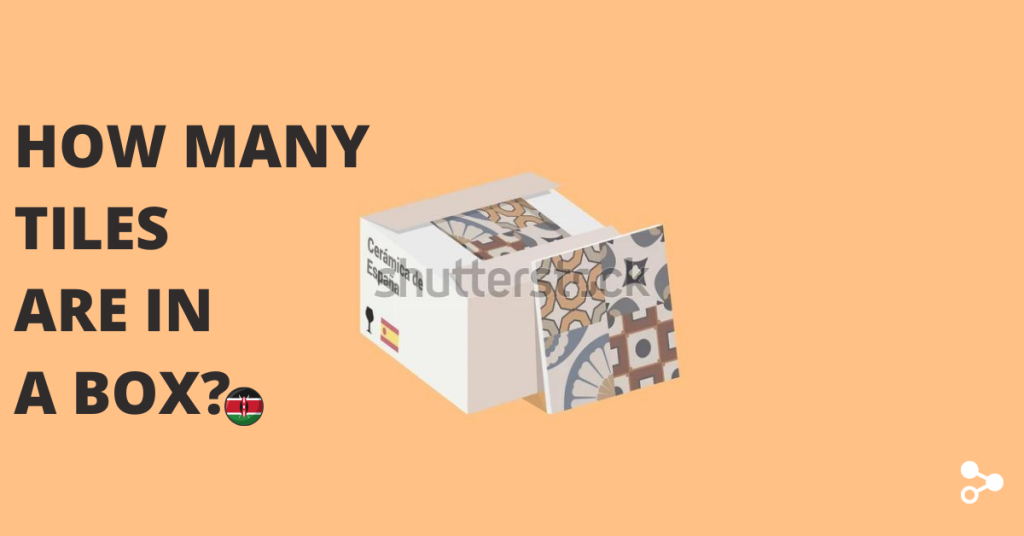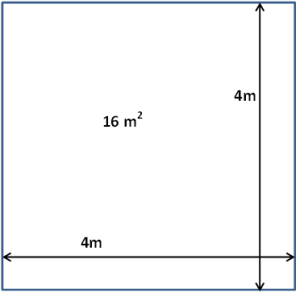# How Many Tiles are in a Box in Kenya

Share to

## How many tiles are in a box?

A box of tiles usually has between 4-25 pieces depending on manufacturer, size of a single tile and type of tile.

Most of the times, the larger each individual tiles is, the less pieces come in a carton. Some boxes with porcelain tiles come with only two pieces and that is why why type of tiles matters.

The following is an example of twyford tiles number of tiles in a box

## Table 1: Number of different tiles dimensions per box

Tiles DimensionNumber of Tiles per box
20cm x 30cm25
25cm x 40cm15
30cm x 30cm17
40cm x 40cm12
60cm x 60cm4

Important: When budgeting your tiling costs, your focus should be on the cost per square meter## Table 2: Cost of common types of tiles in Ksh per square meter

Type of tileCost per square meter (Ksh)
Ceramic700 - 3500
Porcelain1500 - 3000
Granite1700 - 4500
Marble1700 - 4500
Quartzite1700 - 4500
Pebble & stone tiles2000 - 3500

## What area does one box cover?

In order to calculate the area covered by a box of tiles:

• Calculate the area covered by each tile.
• Convert the dimensions to meters then multiply the two sides by each other. For example, 20cm x 30cm tiles would be 0.06 square meters (0.2m x 0.3m).
• Multiply the area per tile by the number of tiles in the carton. For example if there are 25 pieces of the tile above in the carton, the total coverage would be 1.5 square meters (0.06 x 25)